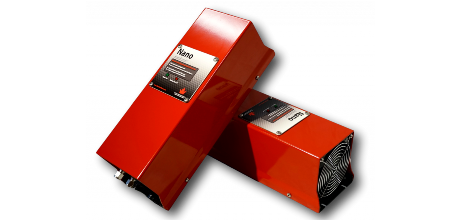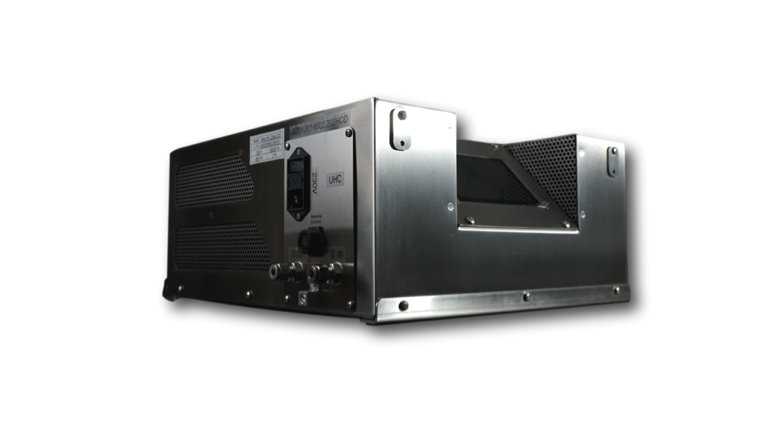## Ozone Library

#### air and soil remediation

Home » Ozone Library » Ozone Conversions and Equations

# Ozone Conversions and Equations

A web resource dedicated to explaining the science behind the main ozone conversion formulas and equations.

## OZONE CONCENTRATION IN WATER:

1 mg/l = 1 PPM O3 = 1 g O3/m3 water {By weight}

## OZONE CONCENTRATION IN AIR BY VOLUME:

1 g O3 / m3 = 467 PPM O3
1 PPM O3 = 2.14 mg O3/m3
100 pphm (parts per hundred million) = 1 ppm (parts per million)

## OZONE CONCENTRATION IN AIR BY WEIGHT:

100 g O3/m3 = 7.8% O3 (Approximate)
1% O3 = 12.8 g O3/m3 (Approximate)
1% O3 = 7,284 PPM Ozone

## OZONE CONCENTRATION IN OXYGEN BY WEIGHT:

100 g O3/m3 = 6.99% O3 (Approximate)
1% O3 = 14.3 g O3/m3 (Approximate)
1% O3 = 6,520 PPM Ozone

## Conversion Table for Ozone Gas Phase Concentration in Oxygen

Weight %Concentration g/m3Productivity at 1 l/min Gas flow g/hr
1 %14.3 g/m³0.86 g/hr
2 %28.7 g/m³1.72 g/hr
4 %57.9 g/m³3.47 g/hr
6 %87.4 g/m³5.24 g/hr
8 % 117.3 g/m³7.04 g/hr
10 %147.70 g/m³8.86 g/hr
12 %178.5 g/m³10.71 g/hr
14 %209.7 g/m³12.58 g/hr
16 %241.3 g/m³14.48 g/hr
18 %273.4 g/m³16.40 g/hr
20 %305.9 g/m³18.36 g/hr
22 %338.9 g/m³20.34 g/hr

## DETERMINING OZONE DOSAGE IN WATER:

The formula is actually very simple.It is:

(water flow rate) x (ozone dosage)= required ozone production

UNITS CONSISTENCY IS VERY IMPORTANT!!!!

Below is the formula for determining ozone generation requirements if you know water flow and ozone dosage (namely flow rate in GPM and ozone dosage in mg/l).

flowrate (GPM) x 3.78 l/gal x 60 min/hr x ozone dosage (mg/l) = ozone production (mg/hr)
Let’s work through an example. How much ozone production is needed to dose 2 PPM into 40 GPM of water? (we will be using PPM throughout the rest of this example knowing that 1 mg/l = 1 PPM)

40 GPM x 3.78 l/gal x 60 min/hr x 2 PPM = 18144 mg/h (18.1 g/h)

Remember that 18 g/h will permit you to dose the water with 2 PPM of ozone. This does not mean that 2 PPM will be your final dissolved ozone concentration if you have a 18g/h generator. Due to efficiency losses with injecting ozone and ozone demand of the water, your dissolved ozone concentration will be less.

## DETERMINE THE OUTPUT OF AN OZONE GENERATOR:

the formula is:

(feed flow rate) x (ozone concentration) = ozone production

Let’s work through an example: The ozone concentration exiting an ozone generator is 120 g/m3 at 5 lpm of oxygen flow. What is the output?

5 l/min x 120 g/m3 x (1 m3/1,000 l) = 0.6 g/min

g/min is not a normal unit in the ozone industry so we simply convert minutes to hours to get g/hr: 0.6 g/min x 60 min/hr = 36 g/hr

## SAMPLE CONVERSIONS:

Convert 140 g/m3 to wt% (oxygen feed gas).

based on the conversion above, 100 g/m3 = 6.99 wt. %

therefore 140 g/m3 / 100 g/m3 x 6.99 wt. % = 9.8 wt.%

## PHYSICAL PROPERTIES STANDARD CONDITIONS (P= 1013.25 MB, T = 273.3K)

Density of ozone: 2.14 kg/m3.
Density of oxygen: 1.43 kg/m3.
Density of air: 1.29 kg/m3.
Density of water: 1 kg/m3.
Molecular weight of ozone: 48 kg·mol−1.
Molecular weight of oxygen: 32 kg·mol−1.

Have Questions?

Click to see aspects## NANO

Click to see aspects## ATLAS

Click to see aspects

## TITAN

Click to see aspects